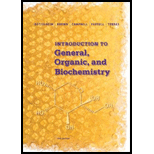# 7-15 A certain reaction is exothermic by 9 kcal/mol and has an activation energy of 14 kcal/mol. Draw an energy diagram for this reaction and label the transition state.### Introduction to General, Organic a...

11th Edition
Frederick A. Bettelheim + 4 others
Publisher: Cengage Learning
ISBN: 9781285869759### Introduction to General, Organic a...

11th Edition
Frederick A. Bettelheim + 4 others
Publisher: Cengage Learning
ISBN: 9781285869759

#### Solutions

Chapter
Section
Chapter 7, Problem 7.15P
Textbook Problem

## 7-15 A certain reaction is exothermic by 9 kcal/mol and has an activation energy of 14 kcal/mol. Draw an energy diagram for this reaction and label the transition state.

Expert Solution
Interpretation Introduction

Interpretation:

An energy diagram for a reaction should be drawn which is exothermic by 9 kcal/mol and has an activation energy of 14 kcal/mol.

Concept Introduction:

Activation energy is the minimum energy which is required to occur a reaction.

Heat of the reaction is the amount of heat absorbed or released during reaction.

An energy level diagram represents a course of reaction. The energy of reactants, transition state and energy of product can be determined from the energy level diagram of a reaction.

Depending on the position of energy of reactant and product in the graph, reaction is classified as endothermic or exothermic.

### Explanation of Solution

In an exothermic reaction, the energy of the reactants is greater than the energy of the products and to form product reactants must go over the activation energy hill as shown.

### Want to see the full answer?

Check out a sample textbook solution.See solution

### Want to see this answer and more?

Bartleby provides explanations to thousands of textbook problems written by our experts, many with advanced degrees!

See solution